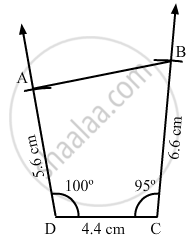Share

# Construct a Quadrilateral Abcd Given Bc = 6.6 Cm, Cd = 4.4 Cm, Ad = 5.6 Cm and ∠D = 100° and ∠C = 95°. - Mathematics

Course
ConceptConstructing a Quadrilateral When Two Adjacent Sides and Three Angles Are Known

#### Question

Construct a quadrilateral ABCD given BC = 6.6 cm, CD = 4.4 cm, AD = 5.6 cm and ∠D = 100° and ∠C= 95°.

#### Solution

Steps of construction:

Step I: Draw DC = 4 . 4 cm .

Step II: Construct ∠ADC = 100° at D .

Step III : With D as the centre and radius 5 . 6 cm, cut off DA = 5 . 6 cm .

Step IV: Construct BCD = 95° at C .

Step V : With C as the centre and radius 6 . 6 cm, cut off CB = 6 . 6 cm .

Step VI: Join AB .